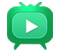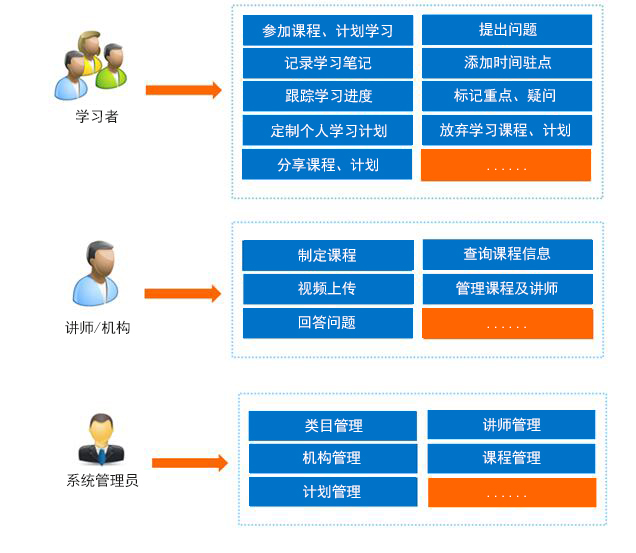﻿ 南京金锟科技有限公司云课堂1.用户端:

X

X

X

X

X

X

X

X

X

X

X

X

X

X

X

X

X

X

X

X

X

X

X
X
X
X

X

X

X

X

X
X
X
X

X
X
X
X

2.管理端:

X
X

X
X

X
X

X
X

X
X

X
X

X
X
X

X
X
X

X
X
X

X
X
X
X

X
X
X
X

X
X
X
X

X
X
X
X

X
X
X

X
X
X
X

X
X
X
X

X
X
X
X

X
X
X
X

X
X
X
X

X
X
X
X

X
X
X
X

X
X
X
X

X
X
X
X